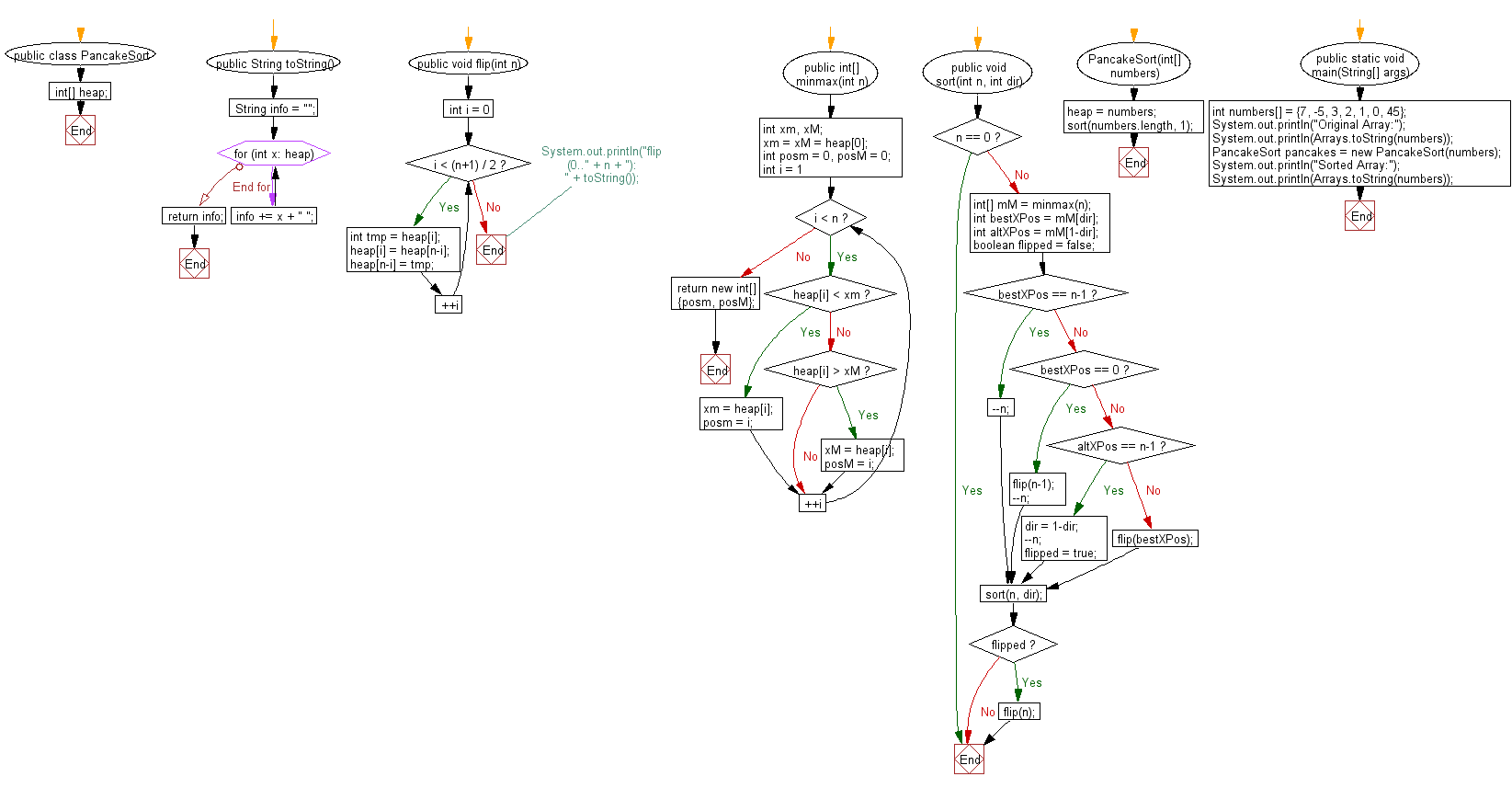﻿ Java exercises: Pancake sort Algorithm - w3resource# Java Exercises: Pancake sort Algorithm

## Java Sorting Algorithm: Exercise-14 with Solution

Write a Java program to sort an array of given integers using Pancake sort Algorithm.

Pancake sorting is the colloquial term for the mathematical problem of sorting a disordered stack of pancakes in order of size when a spatula can be inserted at any point in the stack and used to flip all pancakes above it. A pancake number is the minimum number of flips required for a given number of pancakes. The problem was first discussed by American geometer Jacob E. Goodman. It is a variation of the sorting problem in which the only allowed operation is to reverse the elements of some prefix of the sequence.

Sample Solution:

Java Code:

``````import java.util.Arrays;

public class PancakeSort
{
int[] heap;

public String toString() {
String info = "";
for (int x: heap)
info += x + " ";
return info;
}

public void flip(int n) {
for (int i = 0; i < (n+1) / 2; ++i) {
int tmp = heap[i];
heap[i] = heap[n-i];
heap[n-i] = tmp;
}
// System.out.println("flip(0.." + n + "): " + toString());
}

public int[] minmax(int n) {
int xm, xM;
xm = xM = heap;
int posm = 0, posM = 0;

for (int i = 1; i < n; ++i) {
if (heap[i] < xm) {
xm = heap[i];
posm = i;
}
else if (heap[i] > xM) {
xM = heap[i];
posM = i;
}
}
return new int[] {posm, posM};
}

public void sort(int n, int dir) {
if (n == 0) return;

int[] mM = minmax(n);
int bestXPos = mM[dir];
int altXPos = mM[1-dir];
boolean flipped = false;

if (bestXPos == n-1) {
--n;
}
else if (bestXPos == 0) {
flip(n-1);
--n;
}
else if (altXPos == n-1) {
dir = 1-dir;
--n;
flipped = true;
}
else {
flip(bestXPos);      }
sort(n, dir);

if (flipped) {
flip(n);
}
}

PancakeSort(int[] numbers) {
heap = numbers;
sort(numbers.length, 1);
}

public static void main(String[] args) {
int numbers[] = {7, -5, 3, 2, 1, 0, 45};
System.out.println("Original Array:");
System.out.println(Arrays.toString(numbers));
PancakeSort pancakes = new PancakeSort(numbers);
System.out.println("Sorted Array:");
System.out.println(Arrays.toString(numbers));
}
}
```
```

Sample Output:

```Original Array:
[7, -5, 3, 2, 1, 0, 45]
Sorted Array:
[-5, 0, 1, 2, 3, 7, 45]]
```

Flowchart:Java Code Editor:

What is the difficulty level of this exercise?

﻿

## Java: Tips of the Day

Array vs ArrayLists:

The main difference between these two is that an Array is of fixed size so once you have created an Array you cannot change it but the ArrayList is not of fixed size. You can create instances of ArrayLists without specifying its size. So if you create such instances of an ArrayList without specifying its size Java will create an instance of an ArrayList of default size.

Once an ArrayList is full it re-sizes itself. In fact, an ArrayList is internally supported by an array. So when an ArrayList is resized it will slow down its performance a bit as the contents of the old Array must be copied to a new Array.

At the same time, it's compulsory to specify the size of an Array directly or indirectly while creating it. And also Arrays can store both primitives and objects while ArrayLists only can store objects.

Ref: https://bit.ly/3o8L2KH